# Arduino - Actuator

In this tutorial, we are going to learn:

• How linear actuator works
• How to make linear actuator extend or retract.
• How to control a linear actuator using L298N driver and Arduino.
• How to control the speed of a linear actuator.

This tutorial is for linear actuator without feedback. If you want to learn about linear actuator with feedback, see this Arduino - Actuator with Feedback tutorial.

## Hardware Required

Please note: These are affiliate links. If you buy the components through these links, We may get a commission at no extra cost to you. We appreciate it.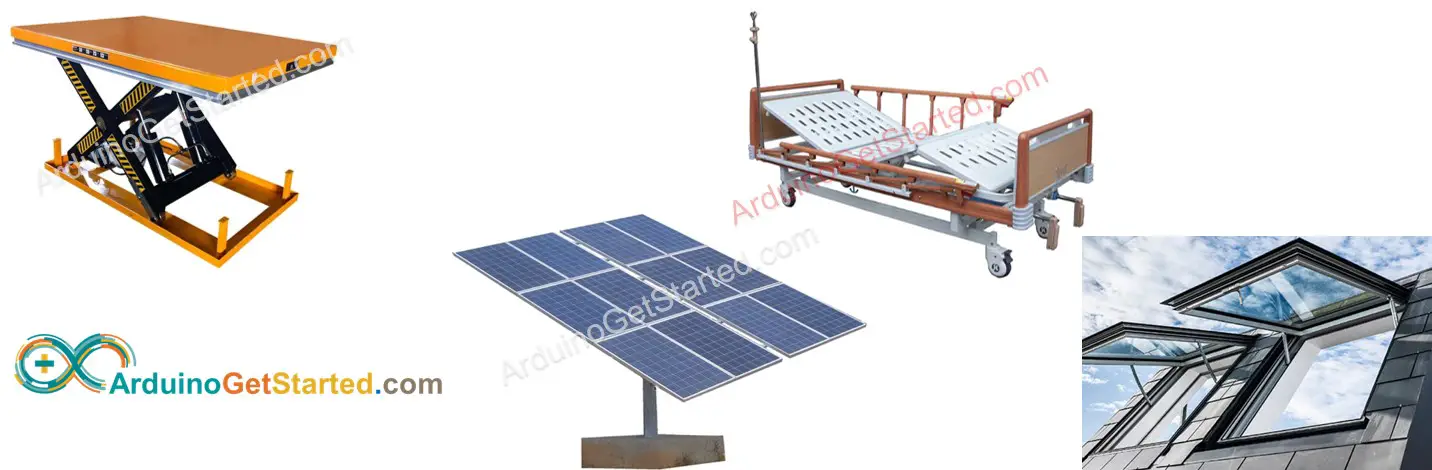### Linear Actuator Pinout

Linear Actuator has two wires:

• Positive wire: usually red
• Negative wire: usually black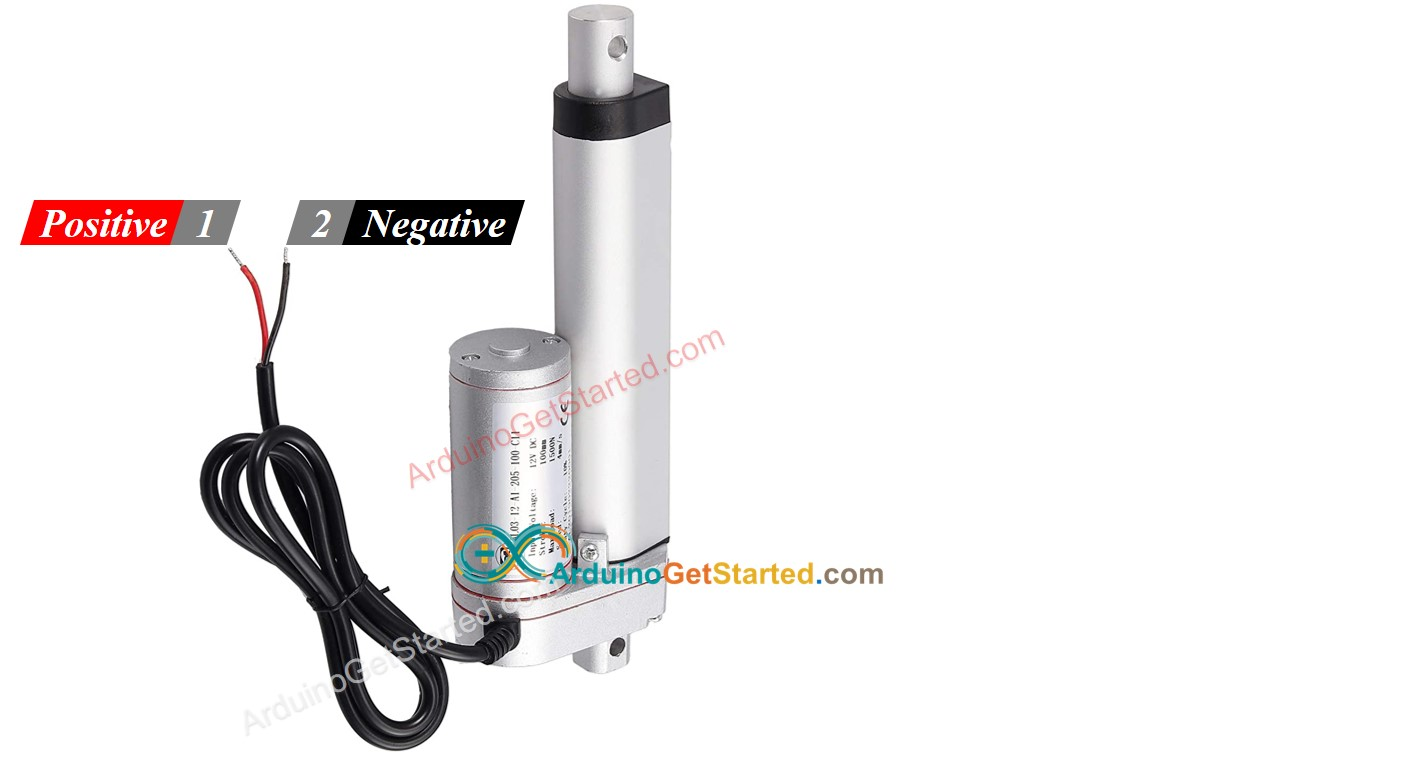### How It Works

When you buy a linear actuator, you need to know what voltage linear actuator works. Let's take a 12V linear actuator as an example.

When you power the 12V linear actuator by a 12V power source:

• 12V and GND to the positive wire and negative wire, respectively: the linear actuator full-speed extends until it reaches the limit.
• 12V and GND to the negative wire and positive wire, respectively: the linear actuator full-speed retracts until it reaches the limit.

While extending or retracting, if we stop power to the actuator (GND to both positive and negative wire), the actuator stops extending/retracting

※ NOTE THAT:

For DC motor, servo motor, and stepper motor without gearing, when carrying a load, if we stop powering, they cannot keep the position. Unlike these motors, the actuator can keep the position even when stopping powering while carrying a load.

If we provide power to linear actuators below 12V, the linear actuator still extends/retracts but not at maximum speed. It means if we change the voltage of the power supply, we can change the speed of the linear actuator. However, this method is not used in practice because of the difficulty in controlling the voltage of the power source. Instead, we fix the voltage of the power source and control the speed of the linear actuator via a PWM signal. The more duty cycle the PWM is, the higher speed the linear actuator extends/retracts.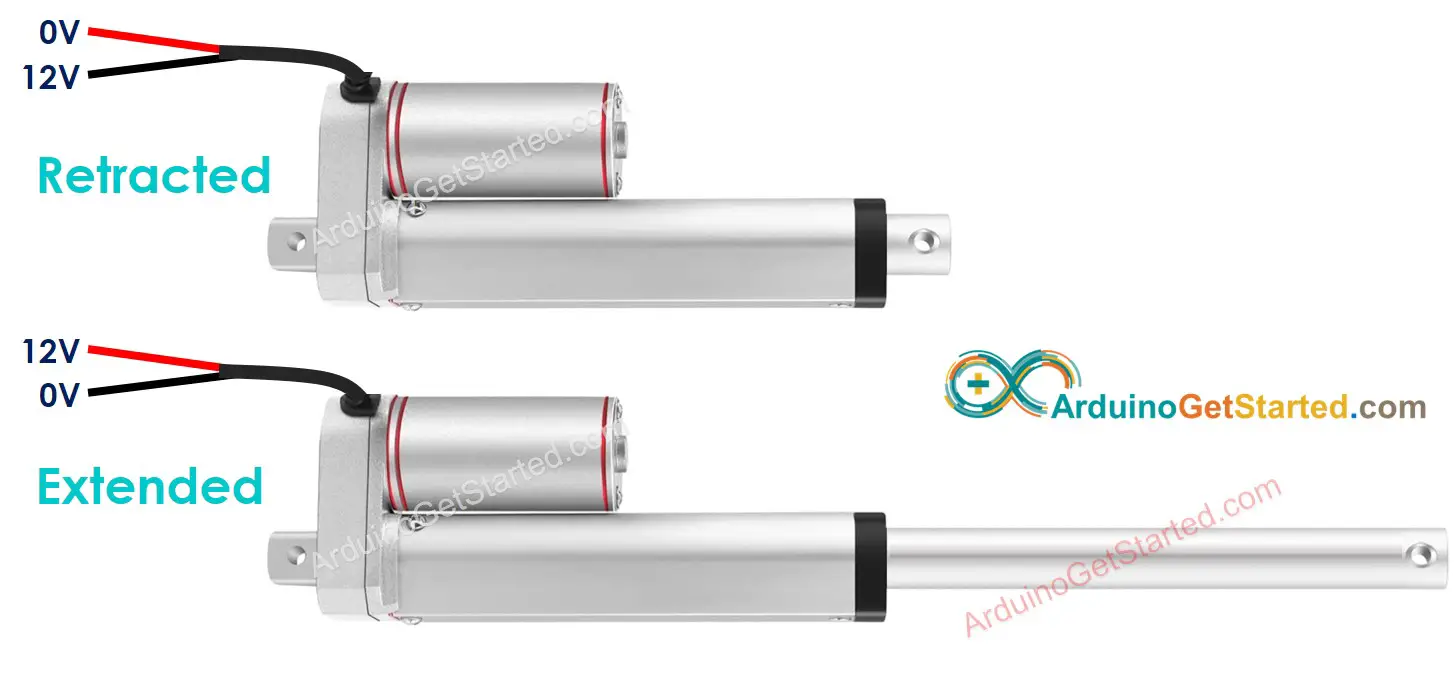### How to control a linear actuator using Arduino

Controlling a linear actuator includes:

• Extends the linear actuator at maximum speed.
• Retracts the linear actuator at maximum speed.
• (optional) controls the extending/retracting speed

Arduino can generate the signal to control the linear actuator. However, this signal has low voltage and current, We cannot use it to control the linear actuator. We need to use a hardware driver in between Arduino and linear actuator. The driver does two works:

• Amplify the control signal from Arduino (current and voltage)
• Receive the another control signal from Arduino to swap pole of power supply → for direction control.

https://arduinogetstarted.com/images/tutorial/arduino-control-linear-actuator.jpg

※ NOTE THAT:

• This tutorial can be applied to all linear actuators. 12V linear actuator is just an example.
• When you controls 5V linear actuator, although Arduino pin outputs 5V (the same as linear actuator voltage), you still needs a driver in between Arduino and linear actuator because the Arduino pin does not provide enough the current for linear actuator.

There are many kinds of the chip, modules (e.g. L293D, L298N) can be used as linear actuator drivers. In this tutorial, we will use the L298N driver.

※ NOTE THAT:

You can also use relays as a driver. However it requires 4 relays to control a single linear actuator (both extend/retract)

L298N Driver can be used to control linear actuator, DC motor and stepper motor. In this tutorial, we learn how to use it to control the linear actuator.### L298N Driver Pinout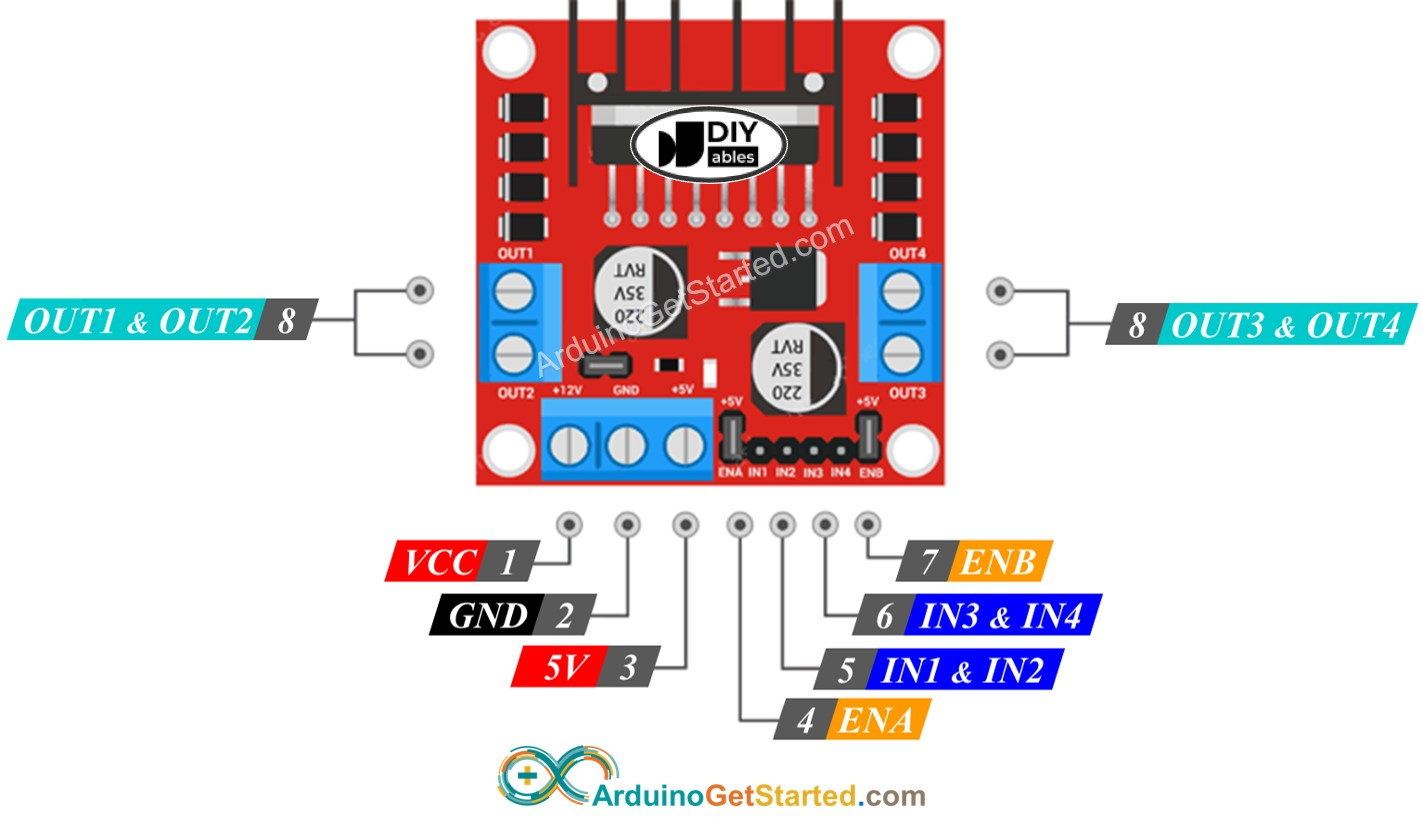L298N Driver has two channels, called channel A and channel B. Therefore, L298N Driver can control two linear actuator independently at the same time. Let's assum that the linear actuator A is connected to channel A, the linear actuator B is connected to channel B. L298N Driver has 13 pins:

#### The common pins for both channels:

• VCC pin: supplies power for the linear actuator. It can be anywhere between 5 to 35V.
• GND pin: is a common ground pin, needs to be connected to GND (0V).
• 5V pin: supplies power for L298N module. It can be supplied by 5V from Arduino.

#### Channel A pins:

• ENA pins: are used to control the speed of the linear actuator A. Removing the jumper and connecting this pin to PWM input will let us control the extending/retracting speed of the linear actuator A.
• IN1 & IN2 pins: are used to control the moving direction of a linear actuator. When one of them is HIGH and the other is LOW, the a linear actuator will extend or retract. If both the inputs are either HIGH or LOW the linear actuator will stop.
• OUT1 & OUT2 pins: are connected to a linear actuator A.

#### Channel B pins:

• ENB pins: are used to control the speed of the linear actuator B. Removing the jumper and connecting this pin to PWM input will let us control the extending/retracting speed of the linear actuator B.
• IN3 & IN4 pins: are used to control the moving direction of a linear actuator. When one of them is HIGH and the other is LOW, the a linear actuator will extend or retract. If both the inputs are either HIGH or LOW the linear actuator will stop.
• OUT3 & OUT4 pins: are connected to a linear actuator.

As described above, the L298N driver has two input powers:

• One for linear actuator (VCC and GND pins): from 5 to 35V.
• One for the L298N module's internal operation (5V and GND pins): from 5 to 7V.

The L298N driver also has three jumpers for advanced uses or other purposes. For the sake of simplicity, please remove all jumpers from the L298N driver.

We can control two linear actuators independently at the same time by using an Arduino and an L298N Driver. To control each linear actuator, we need only three pins from Arduino.

※ NOTE THAT:

The rest of this tutorial controls a linear actuator using channel A. Controlling the other linear actuator is similar.

## How To Control Linear Actuator

We will learn how to control Linear Actuator using L298N driver

### Wiring Diagram

Please remove all three jumpers on the L298N module before wiring.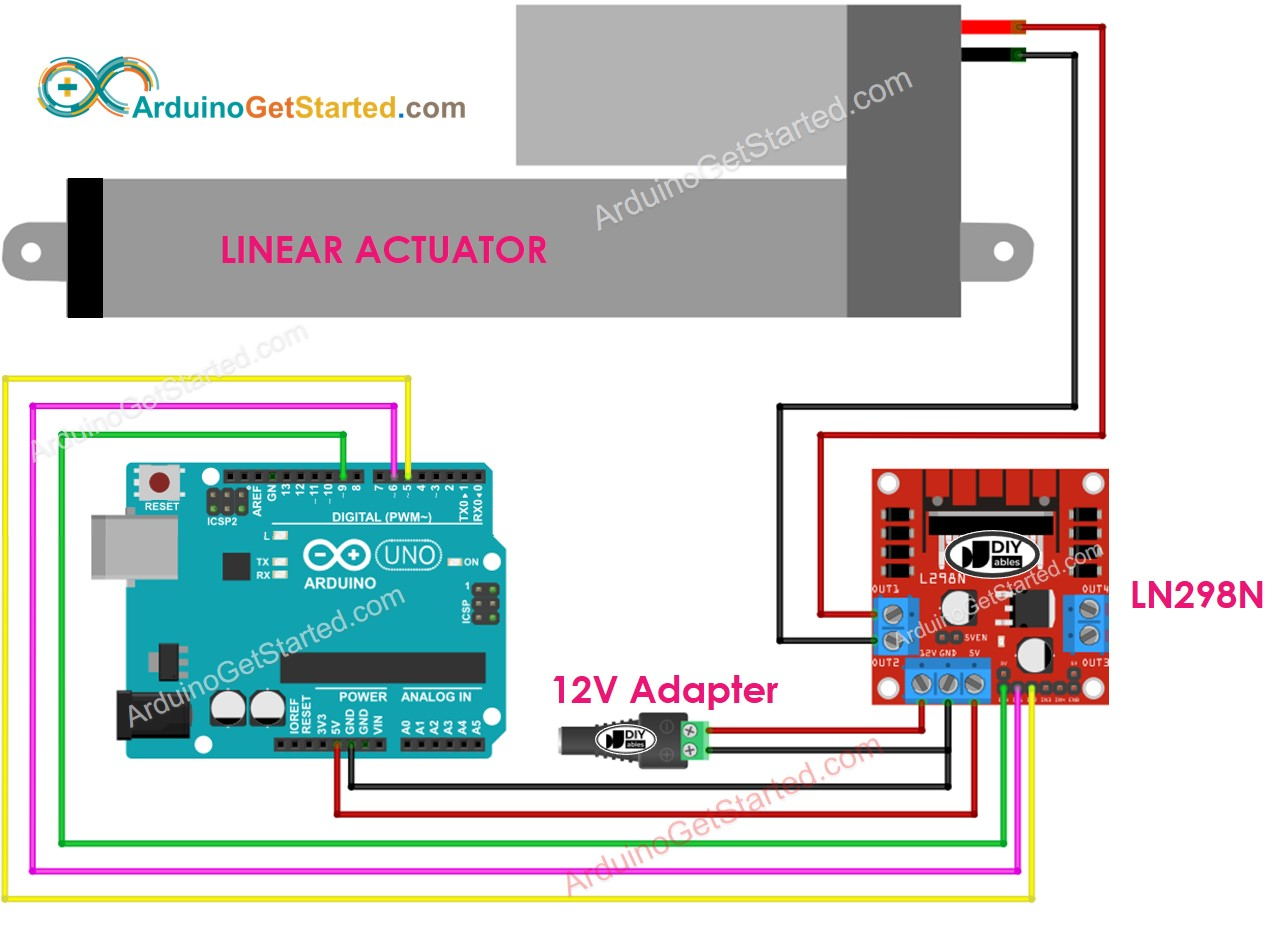This image is created using Fritzing. Click to enlarge image

### How To Make Linear Actuator Expand/Retract

The moving direction of a Linear Actuator can be controlled by applying a logic HIGH/LOW to IN1 and IN2 pins. The below table illustrates how to control the direction in both channels.

IN1 pin IN2 pin Direction
LOW LOW Linear Actuator A stops
HIGH HIGH Linear Actuator A stops
HIGH LOW Linear Actuator A extends
LOW HIGH Linear Actuator A retracts
• Extends Linear Actuator A
digitalWrite(IN1_PIN, HIGH); digitalWrite(IN2_PIN, LOW);
• Retracts Linear Actuator A
digitalWrite(IN1_PIN, LOW); digitalWrite(IN2_PIN, HIGH);

※ NOTE THAT:

The moving direction is inverted if the OUT1 & OUT2 pin connects to two pins of the linear actuator in an inverse way. If so, it just needs to swap between OUT1 & OUT2 pin or change the control signal on IN1 and IN2 pin in the code.

### How To Stop Linear Actuator from Extending or Retracting

The linear actuator automatically stops extending/retracting when it reaches the limit. We can also programmably stop it from extending/retracting while it has not reached the limit.

There are two ways to stop linear actuator

• Controls the speed to 0
analogWrite(ENA_PIN, 0);
• Controls IN1 IN2 pins to the same value (LOW or HIGH)
digitalWrite(IN1_PIN, LOW); digitalWrite(IN2_PIN, LOW);
• Or
digitalWrite(IN1_PIN, HIGH); digitalWrite(IN2_PIN, HIGH);

### How To Control the Speed of Linear Actuator via L298N Driver

It is simple to control the speed of the linear actuator. Instead of controlling ENA pin to HIGH, We generate a PWM signal to the ENA pin. We can do this by:

• Connect an Arduino pin to ENA of L298N
• Generate PWM signal to ENA pin by using analogWrite() function. L298N Driver amplify PWM signal to linear actuator
analogWrite(ENA_PIN, speed); // speed is a value from 0 to 255

The speed is a value between 0 and 255. If the speed is 0, the linear actuator stops. If the speed is 255, the linear actuator extends/retracts at maximum speed.

## Arduino Example Code

The below code does:

• Extend actuator at maximum speed
• Stop the linear actuator
• Retract actuator at maximum speed
• Stop the linear actuator
/* * Created by ArduinoGetStarted.com * * This example code is in the public domain * * Tutorial page: https://arduinogetstarted.com/tutorials/arduino-actuator */ // constants won't change const int ENA_PIN = 9; // the Arduino pin connected to the EN1 pin L298N const int IN1_PIN = 6; // the Arduino pin connected to the IN1 pin L298N const int IN2_PIN = 5; // the Arduino pin connected to the IN2 pin L298N // the setup function runs once when you press reset or power the board void setup() { // initialize digital pins as outputs. pinMode(ENA_PIN, OUTPUT); pinMode(IN1_PIN, OUTPUT); pinMode(IN2_PIN, OUTPUT); digitalWrite(ENA_PIN, HIGH); } // the loop function runs over and over again forever void loop() { // extend the actuator digitalWrite(IN1_PIN, HIGH); digitalWrite(IN2_PIN, LOW); delay(20000); // actuator will stop extending automatically when reaching the limit // retracts the actuator digitalWrite(IN1_PIN, LOW); digitalWrite(IN2_PIN, HIGH); delay(20000); // actuator will stop retracting automatically when reaching the limit }

#### Quick Steps

• Remove all three jumpers on the L298N module.
• Copy the above code and open with Arduino IDE
• Click Upload button on Arduino IDE to upload code to Arduino
• You will see:
• Linear actuator extends and then stops when reaching the limit
• Linear actuator keeps the position a mount of time
• Linear actuator retracts and then stops when reaching the limit
• Linear actuator keeps the position a mount of time
• The above process is run repeatedly.

## Video Tutorial

We are considering to make the video tutorials. If you think the video tutorials are essential, please subscribe to our YouTube channel to give us motivation for making the videos.